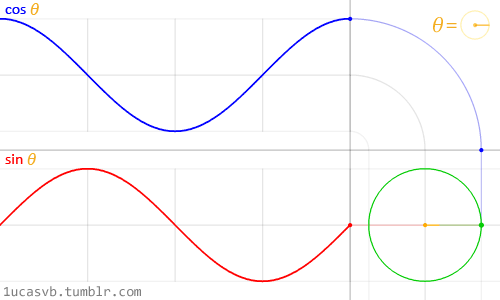# What can I do with these asin, acos, atan, sin, cos, tan

You can write your topic however you want, but you need to answer these questions:

1. What do you want to achieve? Keep it simple and clear!

Wants to use all their potentials

1. What is the issue? Include screenshots / videos if possible!

I’m bad at math and I don’t understand everything.

1. What solutions have you tried so far? Did you look for solutions on the Developer Hub?

I tried with Youtube, but its very hard.

A few exemples are enough, thanks.

4 Likes

Once you start to get into Algebra or Trigonometry classes, you’ll understand how these work; until then, I wouldn’t recommend you use them as you wouldn’t understand them. You might be able to find some Trigonometry tutorials online that help explain these, however.

3 Likes

Yes, i understand it a bit, but how to use sin, cos and tan?

But i dont understand the asin, acos and atan.

Like I said, once you get into higher level Maths classes, you’ll understand how these work. I’d recommend using Khan Academy; it’s a pretty decent source to learn Maths from, especially Trigonometry.

3 Likes

Those are trigonometric functions. The use cases are as plenty as there are use cases for addition and subtraction. You will most likely learn these functions in a trig or pre-calculus class.

Figure A:

Figure B:Here I’m just going to talk about sine and cosine + their inverse functions asin and acos (`math.sin` and `math.cos` + `math.asin` and `math.acos`). It’s helpful to think about sine and cosine as the Y and X coordinates on a plane as Θ (theta) increases. In figure B, you can see the graph of `y = sin(Θ)` depicted by the red line and the graph of `x = cos(Θ)` in blue. It also shows you how that translates into coordinates on a plane. If you calculate x and y for every number between 0 and 2 * pi, it will result in a circle! You can graph the functions `y=cos(x)` and `y=sin(x)` from 0 to 2π on Desmos and convert the two values into X and Y coordinates if figure is confusing to you.

Tangent is the ratio between sine and cosine `tan(Θ) = sin(Θ) / cos(Θ)`. Often times it can be represented as the “slope”; the “instantaneous tangent line” is something you learn how to calculate in a calculus class with limits and derivatives. Don’t worry too much about this one until you grasp the concept of sine and cosine first.

`math.asin` and `math.acos` do the opposite (inverse) of `math.sin` and `math.cos`. They return the Θ value that corresponds to the number from -1, 1 you pass in. I wouldn’t worry too much about those right now though. If you have any questions, feel free to ask them here.

23 Likes

If you’re really committed, Khan Academy has a free course on trig.

Learning things like this for ur Roblox projects is awesome when compared to learning things like this for a grade in school.

3 Likes

Thank you all, but I have to go to sleep net unfortunately, tomorrow I will ask all the questions I have in mind. Good night!

1 Like

Can you explain me this:

``````  local rDist = (camCF.p - surfaceCF.p):Dot(surfaceCF.LookVector)
``````

The link I found this script in is this one

I was once just like you, not understanding any scripting or coding but after 4 years of algebra, i finally understood it. Now I’m a pro. Don’t worry, it does get easier with experience.

2 Likes

I use them most in springing and camera bobbing.

I would need to see the code in context. What is the code from? Can you send pictures?

1 Like

HERE, see Post 19

Thank you really for all your help, but one more question remains, is it really true that you would rather use the sine for the Y axis and the cosine for the X axis, if so, does that mean that the tangent is used for the Z axis? (P.S.: I saw it in this video, time `2:36`)

2 Likes

It’s called the dot product, in vector calculus you use it to get the angle between two vectors.

5 Likes